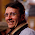## Thursday, May 26, 2011

### A nice DP problem

Given an array A of positive integers. Convert it to a sorted array with minimum cost. The only valid operation are:
1) Decrement with cost = 1
2) Delete an element completely from the array with cost = value of element

Any solution? convert here means you can get a different sequence, but it must be non decreasing.

A possible solution is with DP. C(n, m) = is the cost of getting n non decreasing elements with the constrain tha no element is greater than m. note that given the # cardinality of the set made up of the elements in #set(A), then 0 < m < #set(A)

```C(1,m) = max(a - m, 0)  // because at first step you can only decrease of  value m
C(n, m) =  min (   // min: two options and want to find the min cost

a[n] + C(n - 1, m), // delete operation at step n for value m
// express the cost in function of step n-1 for value m```
```// and sum the cost of the specific delete op as specified
```
`// decrement`
`(a[n] <= m) ?       C(n - 1, a[n]) // there is no need to decrement but for having// a non decreasing sequence we must have a value// non larger than a[n] for a[1..n-1]. That is // expressed inductively by C(n-1, a[n]):        C(n-1, m)  +   // decrement with cost ma[n]-  m       // cost of decrementing a[n] to m with a[n] - m ops`
```)

```

1.The solution you presented is O(n^2).

One can speed it up to O(n log n) by taking a closer look at what you do for a fixed value of n and all values of m.

1. Let m0 be the smallest m, such that C(n-1,m)=c.

An adding interval tree that holds a value (ax+b) and the maximum in each node seems to be a good candidate.

1a is logarithmic - compute and add up values on the way to the root.
1b is logarithmic - binary search by max.
2 and 4 are logarithmic - update value for O(log n) nodes and recompute max for O(log n) nodes.
3 is amortised logarithmic - like 2 and 4, but we need to clean up. However, we only clean up as much as we've previously made dirty (i.e. nodes with max>0).

I don't know if there exists an O(n) solution. I suspect not.

2.why you see it as O(n^2)?

3.By n, I meant the length of A. Sorry for not mentioning that earlier.

I imagine, that you want to read the answer to the original puzzle by taking the minimum of C(n,m) for all possible values of m. The formula you presented requires at least computing C(n-1,m) in order to that. Therefore, at some point you have to compute C(k,m) for k between 1 and n and all relevant m.

One can prove that it suffices to consider m among the values of A and there's at most n of them. So you have at most n^2 C(k,m) values to compute, each in O(1) time. That's where I got the O(n^2) from.

But maybe I am under/over-interpreting something here. Apologies in advance.